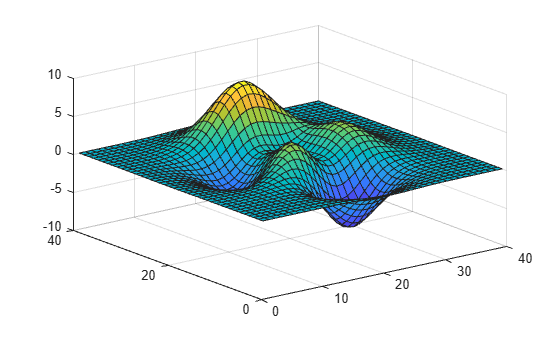# cputime

CPU time used by MATLAB

## Syntax

``t = cputime``

## Description

example

````t = cputime` returns the total CPU time used by MATLAB® since it was started. The returned CPU time is expressed in seconds.Each call to `cputime` returns the total CPU time used by MATLAB up to the point when the function is called. To measure the CPU time used to run your code, place two calls to `cputime` before and after the code, and then calculate the difference between the returned values.```

## Examples

collapse all

Measure the CPU time used to run `surf(peaks(40))`.

```tStart = cputime; surf(peaks(40))````tEnd = cputime - tStart`
```tEnd = 0.3700 ```

## Tips

• To measure the performance of your code, use the `timeit` or `tic` and `toc` functions. Unlike the `cputime` function, which measures CPU time, `timeit` or `tic/toc` return wall-clock time.

For example, the CPU time for `pause` is typically small:

```tStart = cputime; pause(1) tEnd = cputime - tStart ```
```tEnd = 0.1094 ```

However, the wall-clock time accounts for the actual time that MATLAB execution is paused:

```tic pause(1) toc```
```Elapsed time is 1.000483 seconds. ```